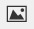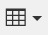PSABM6M8 - Student Facing Task---Acc7.3 Lesson 5 Reasoning about Equations and Tape Diagrams (Part 2) (7.EE.B.3, 7.EE.B.4, 7.EE.B.4.a)
Part A)

Solve each equation mentally.

x - 1 = 5

x = _____

Type your answer below as a number (example: 5, 3.1, 4 1/2, or 3/2):
Part B)

2(x - 1) = 10

x = _____

Type your answer below as a number (example: 5, 3.1, 4 1/2, or 3/2):
Part C)

3(x - 1) = 15

x = _____

Type your answer below as a number (example: 5, 3.1, 4 1/2, or 3/2):
Part D)

500 = 100(x - 1)

x = _____

Type your answer below as a number (example: 5, 3.1, 4 1/2, or 3/2):
Part A)

Draw a tape diagram to represent each situation. For some of the situations, you need to decide what to represent with a variable.

Each of 5 gift bags contains x pencils. Tyler adds 3 more pencils to each bag. Altogether, the gift bags contain 20 pencils.

Draw your tape diagram on a piece of paper, take a picture of it, and upload the image using the image upload icon.  Alternatively, You can make a tape diagram using the table button.

Part B)

Noah drew an equilateral triangle with sides of length 5 inches. He wants to increase the length of each side by x inches so the triangle is still equilateral and has a perimeter of 20 inches.

Draw your tape diagram on a piece of paper, take a picture of it, and upload the image using the image upload icon.  Alternatively, You can make a tape diagram using the table button.

Part C)

An art class charges each student \$3 to attend plus a fee for supplies. Today, \$20 was collected for the 5 students attending the class.

Draw your tape diagram on a piece of paper, take a picture of it, and upload the image using the image upload icon.  Alternatively, You can make a tape diagram using the table button.

Part D)

Elena ran 20 miles this week, which was three times as far as Clare ran this week. Clare ran 5 more miles this week than she did last week.

Draw your tape diagram on a piece of paper, take a picture of it, and upload the image using the image upload icon.  Alternatively, You can make a tape diagram using the table button.

Part A)

Match each situation to an equation.  The situations are as follows:

1: Each of 5 gift bags contains x pencils. Tyler adds 3 more pencils to each bag. Altogether, the gift bags contain 20 pencils.

2: Noah drew an equilateral triangle with sides of length 5 inches. He wants to increase the length of each side by x inches so the triangle is still equilateral and has a perimeter of 20 inches.

3: An art class charges each student \$3 to attend plus a fee for supplies. Today, \$20 was collected for the 5 students attending the class.

4: Elena ran 20 miles this week, which was three times as far as Clare ran this week. Clare ran 5 more miles this week than she did last week.

Each situation in the previous activity is represented by one of the following equations.

(x + 3) * 5 = 20

3(x + 5) = 20

Check all the situations that match (x + 3) * 5 = 20, and leave all the situations that match 3(x + 5) = 20 unchecked.

Select all that apply:
Part B)

Find the solution to (x + 3) * 5  = 20. Use your diagrams to help you reason.

x = _____

Type your answer below as a number (example: 5, 3.1, 4 1/2, or 3/2):
Part C)

x = ______

Type your answer below as a number (example: 5, 3.1, 4 1/2, or 3/2):
Part D)

What does each solution tell you about its situation?